# Propositional and FirstOrder Logic Chapter 7 47 8

• Slides: 36Propositional and First-Order Logic Chapter 7. 4─7. 8, 8. 1─8. 3, 8. 5 Some material adopted from notes by Andreas Geyer-Schulz and Chuck DyerLogic roadmap overview • Propositional logic (review) • Problems with propositional logic • First-order logic (review) – Properties, relations, functions, quantifiers, … – Terms, sentences, wffs, axioms, theories, proofs, … • Extensions to first-order logic • Logical agents – Reflex agents – Representing change: situation calculus, frame problem – Preferences on actions – Goal-based agentsDisclaimer “Logic, like whiskey, loses its beneficial effect when taken in too large quantities. ” - Lord Dunsany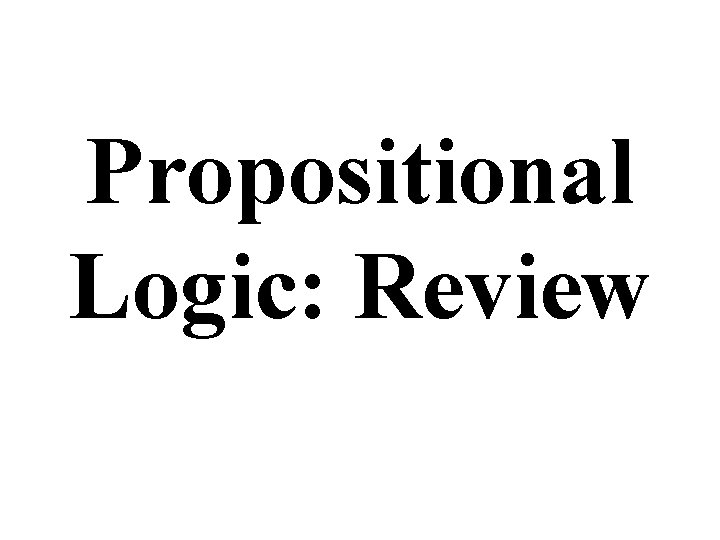Propositional Logic: ReviewBig Ideas • Logic is a great knowledge representation language for many AI problems • Propositional logic is the simple foundation and fine for some AI problems • First order logic (FOL) is much more expressive as a KR language and more commonly used in AI • There are many variations: horn logic, higher order logic, three-valued logic, probabilistic logics, etc.Propositional logic • Logical constants: true, false • Propositional symbols: P, Q, . . . (atomic sentences) • Wrapping parentheses: ( … ) • Sentences are combined by connectives: and [conjunction] or [disjunction] implies [implication / conditional] is equivalent[biconditional] not [negation] • Literal: atomic sentence or negated atomic sentence P, PExamples of PL sentences • (P Q) R “If it is hot and humid, then it is raining” • Q P “If it is humid, then it is hot” • Q “It is humid. ” • We’re free to choose better symbols, btw: Ho = “It is hot” Hu = “It is humid” R = “It is raining”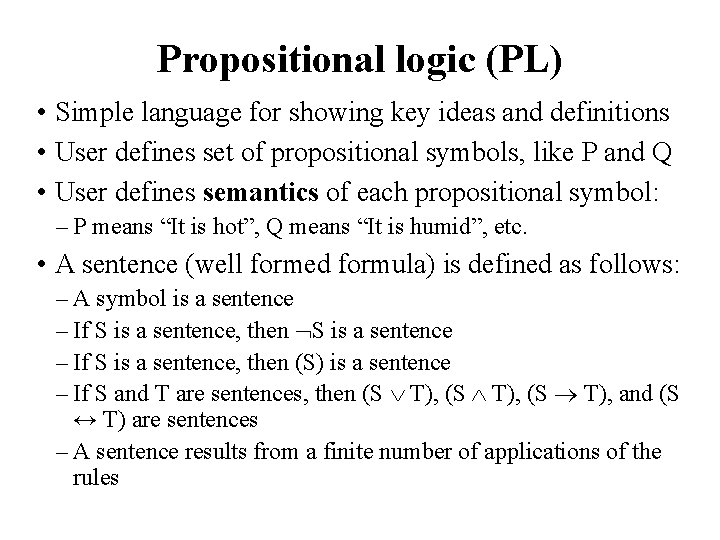Propositional logic (PL) • Simple language for showing key ideas and definitions • User defines set of propositional symbols, like P and Q • User defines semantics of each propositional symbol: – P means “It is hot”, Q means “It is humid”, etc. • A sentence (well formed formula) is defined as follows: – A symbol is a sentence – If S is a sentence, then S is a sentence – If S is a sentence, then (S) is a sentence – If S and T are sentences, then (S T), and (S ↔ T) are sentences – A sentence results from a finite number of applications of the rulesSome terms • The meaning or semantics of a sentence determines its interpretation • Given the truth values of all symbols in a sentence, it can be “evaluated” to determine its truth value (True or False) • A model for a KB is a possible world – an assignment of truth values to propositional symbols that makes each sentence in the KB True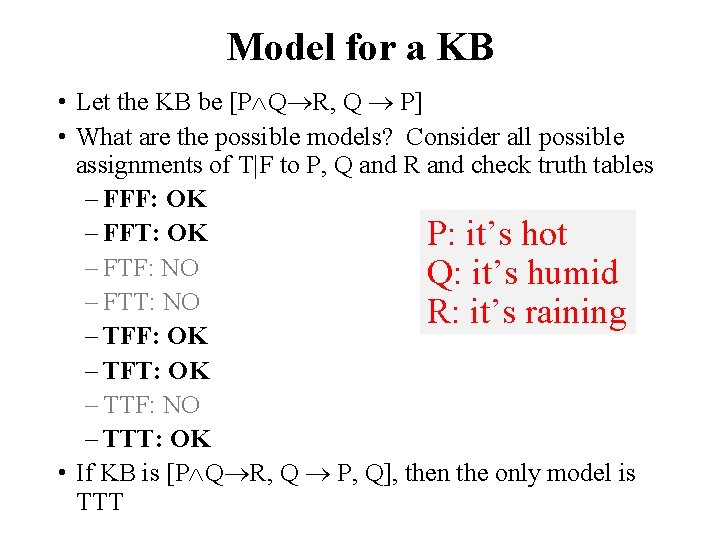Model for a KB • Let the KB be [P Q R, Q P] • What are the possible models? Consider all possible assignments of T|F to P, Q and R and check truth tables – FFF: OK – FFT: OK P: it’s hot – FTF: NO Q: it’s humid – FTT: NO R: it’s raining – TFF: OK – TFT: OK – TTF: NO – TTT: OK • If KB is [P Q R, Q P, Q], then the only model is TTT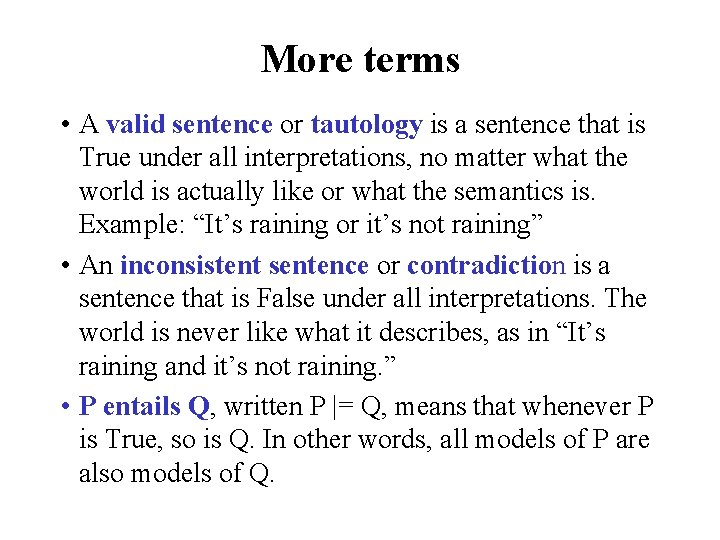More terms • A valid sentence or tautology is a sentence that is True under all interpretations, no matter what the world is actually like or what the semantics is. Example: “It’s raining or it’s not raining” • An inconsistent sentence or contradiction is a sentence that is False under all interpretations. The world is never like what it describes, as in “It’s raining and it’s not raining. ” • P entails Q, written P |= Q, means that whenever P is True, so is Q. In other words, all models of P are also models of Q.Truth tables • Truth tables are used to define logical connectives • and to determine when a complex sentence is true given the values of the symbols in it Truth tables for the five logical connectives Example of a truth table used for a complex sentence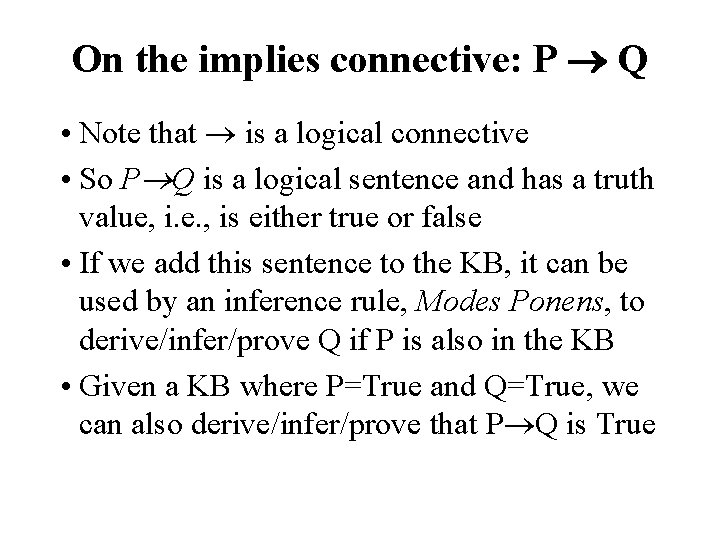On the implies connective: P Q • Note that is a logical connective • So P Q is a logical sentence and has a truth value, i. e. , is either true or false • If we add this sentence to the KB, it can be used by an inference rule, Modes Ponens, to derive/infer/prove Q if P is also in the KB • Given a KB where P=True and Q=True, we can also derive/infer/prove that P Q is True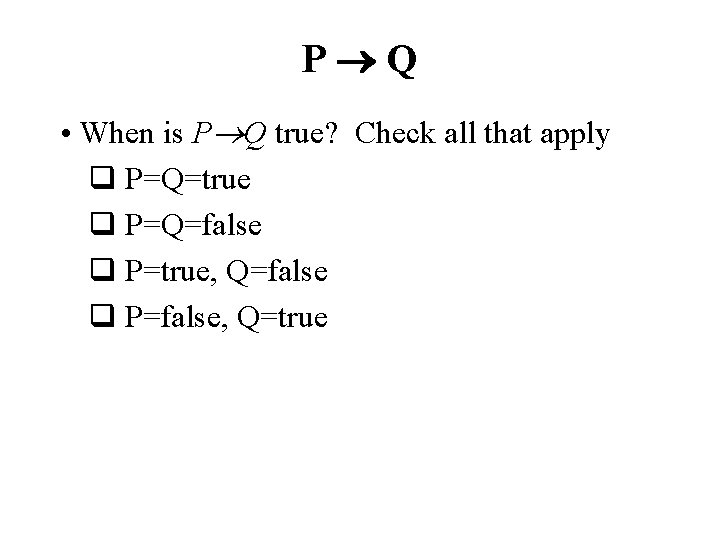P Q • When is P Q true? Check all that apply q P=Q=true q P=Q=false q P=true, Q=false q P=false, Q=trueP Q • When is P Q true? Check all that apply ✔ P=Q=true q ✔ P=Q=false q q P=true, Q=false ✔ P=false, Q=true q • We can get this from the truth table for • Note: in FOL it’s much harder to prove that a conditional true. – Consider proving prime(x) odd(x)Inference rules • Logical inference creates new sentences that logically follow from a set of sentences (KB) • An inference rule is sound if every sentence X it produces when operating on a KB logically follows from the KB – i. e. , inference rule creates no contradictions • An inference rule is complete if it can produce every expression that logically follows from (is entailed by) the KB. – Note analogy to complete search algorithms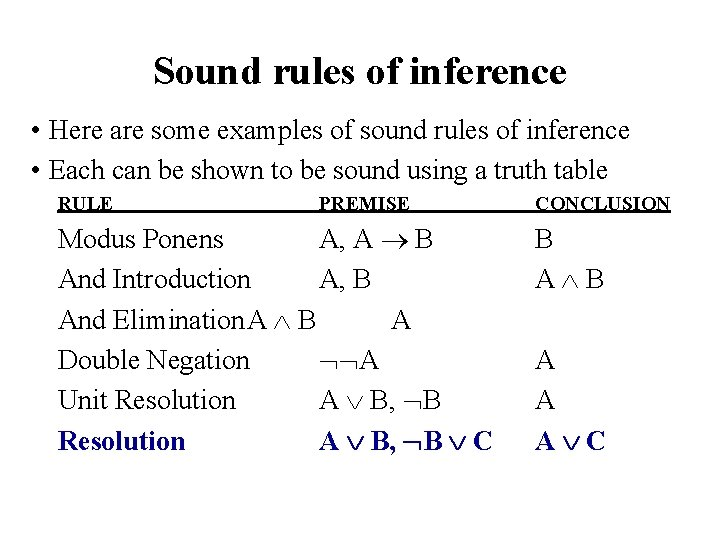Sound rules of inference • Here are some examples of sound rules of inference • Each can be shown to be sound using a truth table RULE PREMISE Modus Ponens A, A B And Introduction A, B And Elimination. A B A Double Negation A Unit Resolution A B, B C CONCLUSION B A A A CSoundness of modus ponens A B A→B OK? True False True False TrueResolution • Resolution is a valid inference rule producing a new clause implied by two clauses containing complementary literals – A literal is an atomic symbol or its negation, i. e. , P, ~P • Amazingly, this is the only interference rule you need to build a sound and complete theorem prover – Based on proof by contradiction and usually called resolution refutation • The resolution rule was discovered by Alan Robinson (CS, U. of Syracuse) in the mid 60 s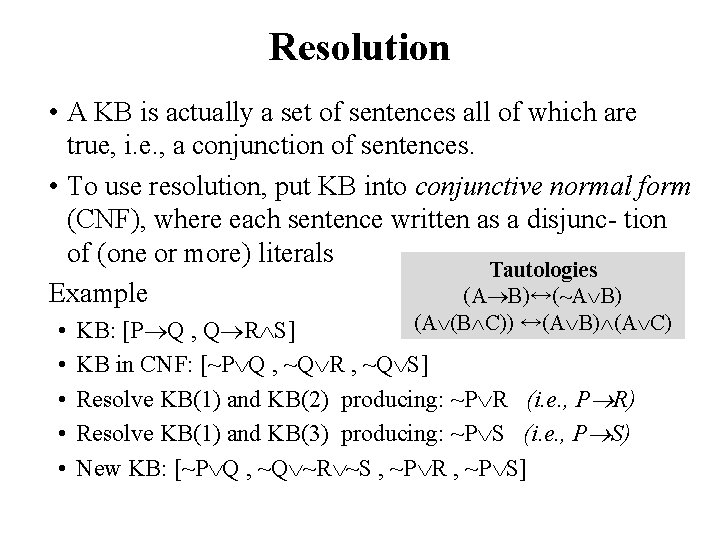Resolution • A KB is actually a set of sentences all of which are true, i. e. , a conjunction of sentences. • To use resolution, put KB into conjunctive normal form (CNF), where each sentence written as a disjunc- tion of (one or more) literals Tautologies Example (A B)↔(~A B) • • • (A (B C)) ↔(A B) (A C) KB: [P Q , Q R S] KB in CNF: [~P Q , ~Q R , ~Q S] Resolve KB(1) and KB(2) producing: ~P R (i. e. , P R) Resolve KB(1) and KB(3) producing: ~P S (i. e. , P S) New KB: [~P Q , ~Q ~R ~S , ~P R , ~P S]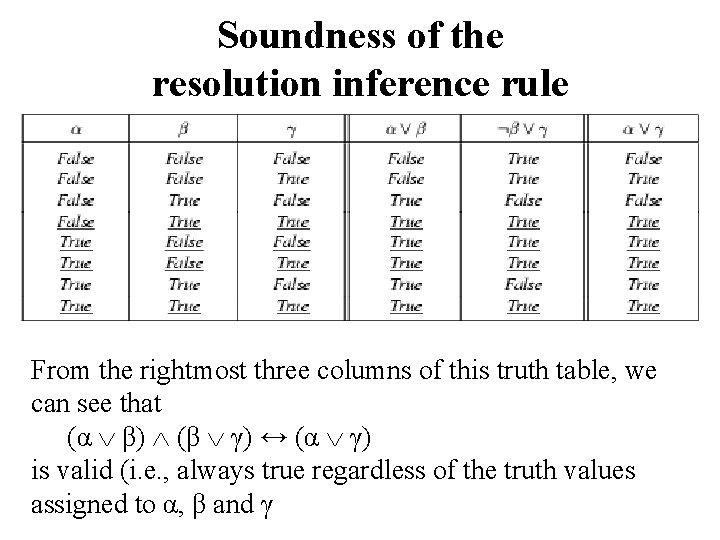Soundness of the resolution inference rule From the rightmost three columns of this truth table, we can see that (α β) (β γ) ↔ (α γ) is valid (i. e. , always true regardless of the truth values assigned to α, β and γ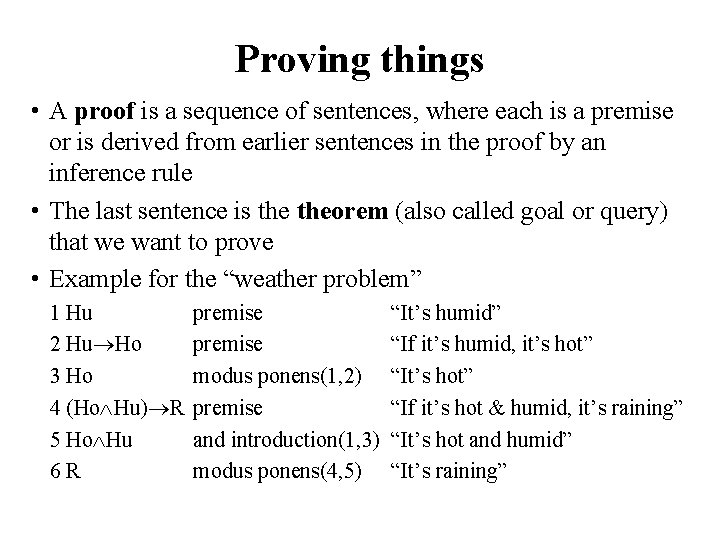Proving things • A proof is a sequence of sentences, where each is a premise or is derived from earlier sentences in the proof by an inference rule • The last sentence is theorem (also called goal or query) that we want to prove • Example for the “weather problem” 1 Hu 2 Hu Ho 3 Ho 4 (Ho Hu) R 5 Ho Hu 6 R premise modus ponens(1, 2) premise and introduction(1, 3) modus ponens(4, 5) “It’s humid” “If it’s humid, it’s hot” “If it’s hot & humid, it’s raining” “It’s hot and humid” “It’s raining”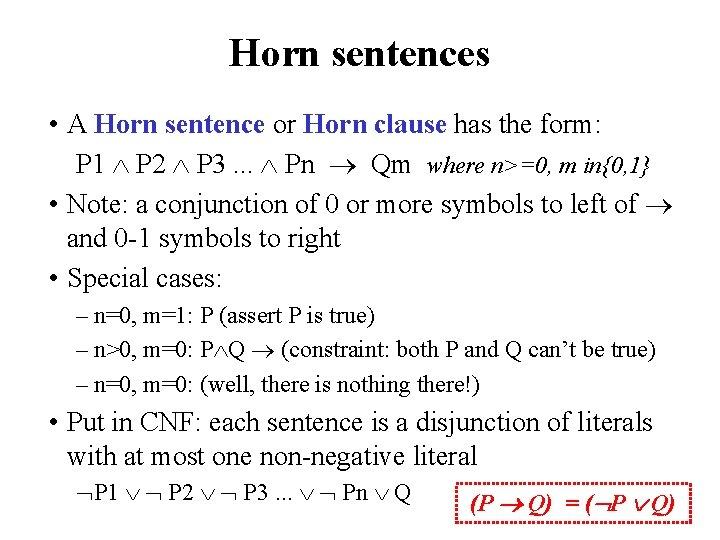Horn sentences • A Horn sentence or Horn clause has the form: P 1 P 2 P 3. . . Pn Qm where n>=0, m in{0, 1} • Note: a conjunction of 0 or more symbols to left of and 0 -1 symbols to right • Special cases: – n=0, m=1: P (assert P is true) – n>0, m=0: P Q (constraint: both P and Q can’t be true) – n=0, m=0: (well, there is nothing there!) • Put in CNF: each sentence is a disjunction of literals with at most one non-negative literal P 1 P 2 P 3. . . Pn Q (P Q) = ( P Q)Significance of Horn logic • We can also have horn sentences in FOL • Reasoning with horn clauses is much simpler – Satisfiability of a propositional KB (i. e. , finding values for a symbols that will make it true) is NP complete – Restricting KB to horn sentences, satisfiability is in P • For this reason, FOL Horn sentences are the basis for Prolog and Datalog • What Horn sentences give up are handling, in a general way, (1) negation and (2) disjunctions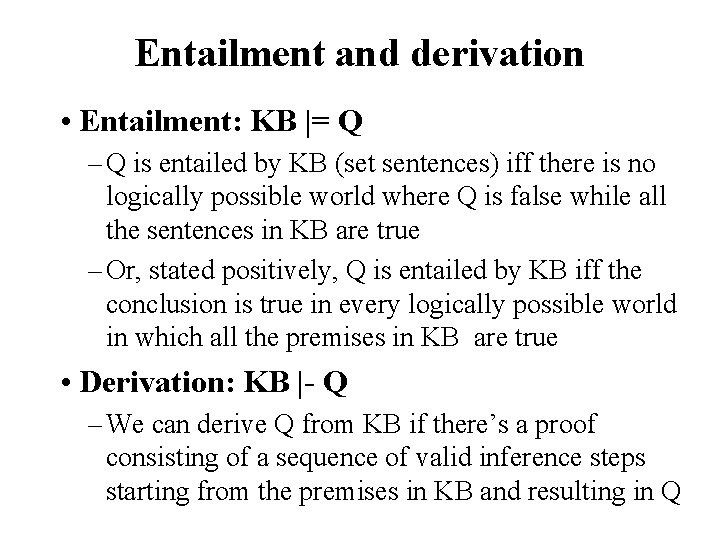Entailment and derivation • Entailment: KB |= Q – Q is entailed by KB (set sentences) iff there is no logically possible world where Q is false while all the sentences in KB are true – Or, stated positively, Q is entailed by KB iff the conclusion is true in every logically possible world in which all the premises in KB are true • Derivation: KB |- Q – We can derive Q from KB if there’s a proof consisting of a sequence of valid inference steps starting from the premises in KB and resulting in QTwo important properties for inference Soundness: If KB |- Q then KB |= Q – If Q is derived from KB using a given set of rules of inference, then Q is entailed by KB – Hence, inference produces only real entailments, or any sentence that follows deductively from the premises is valid Completeness: If KB |= Q then KB |- Q – If Q is entailed by KB, then Q can be derived from KB using the rules of inference – Hence, inference produces all entailments, or all valid sentences can be proved from the premisesProblems with Propositional Logic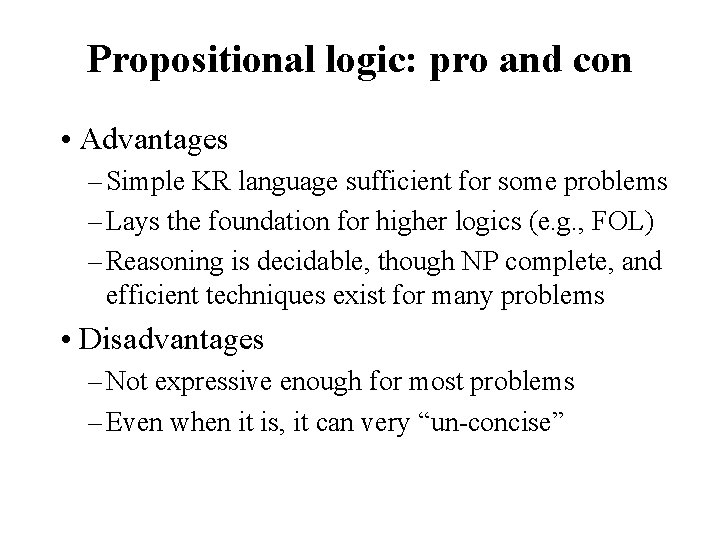Propositional logic: pro and con • Advantages – Simple KR language sufficient for some problems – Lays the foundation for higher logics (e. g. , FOL) – Reasoning is decidable, though NP complete, and efficient techniques exist for many problems • Disadvantages – Not expressive enough for most problems – Even when it is, it can very “un-concise”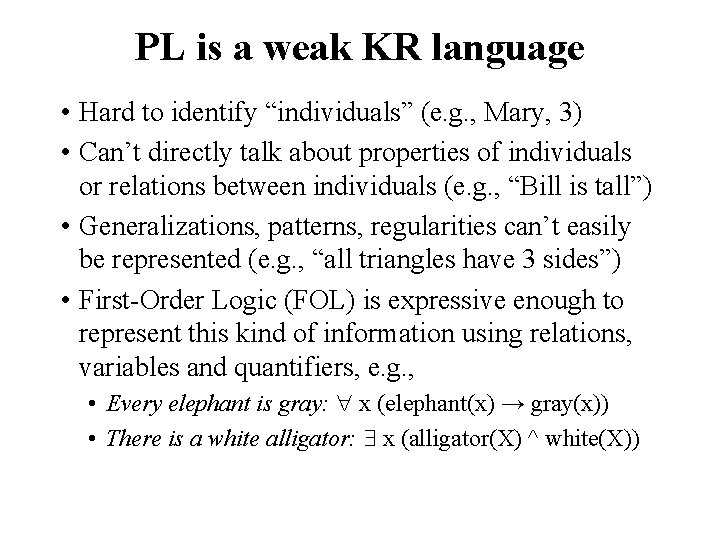PL is a weak KR language • Hard to identify “individuals” (e. g. , Mary, 3) • Can’t directly talk about properties of individuals or relations between individuals (e. g. , “Bill is tall”) • Generalizations, patterns, regularities can’t easily be represented (e. g. , “all triangles have 3 sides”) • First-Order Logic (FOL) is expressive enough to represent this kind of information using relations, variables and quantifiers, e. g. , • Every elephant is gray: x (elephant(x) → gray(x)) • There is a white alligator: x (alligator(X) ^ white(X))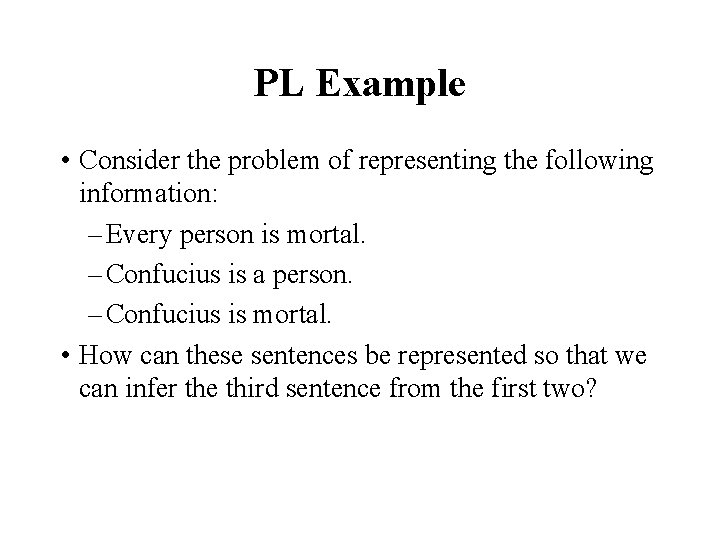PL Example • Consider the problem of representing the following information: – Every person is mortal. – Confucius is a person. – Confucius is mortal. • How can these sentences be represented so that we can infer the third sentence from the first two?PL Example • In PL we have to create propositional symbols to stand for all or part of each sentence, e. g. : P = “person”; Q = “mortal”; R = “Confucius” • The above 3 sentences are represented as: P Q; R P; R Q • The 3 rd sentence is entailed by the first two, but we need an explicit symbol, R, to represent an individual, Confucius, who is a member of the classes person and mortal • Representing other individuals requires introducing separate symbols for each, with some way to represent the fact that all individuals who are “people” are also “mortal”Hunt the Wumpus domain • Some atomic propositions: S 12 = There is a stench in cell (1, 2) B 34 = There is a breeze in cell (3, 4) W 22 = Wumpus is in cell (2, 2) V 11 = We’ve visited cell (1, 1) OK 11 = Cell (1, 1) is safe. … • Some rules: (R 1) S 11 W 11 W 12 W 21 (R 2) S 21 W 11 W 22 W 31 (R 3) S 12 W 11 W 12 W 22 W 13 (R 4) S 12 W 13 W 12 W 22 W 11 … • The lack of variables requires us to give similar rules for each cell!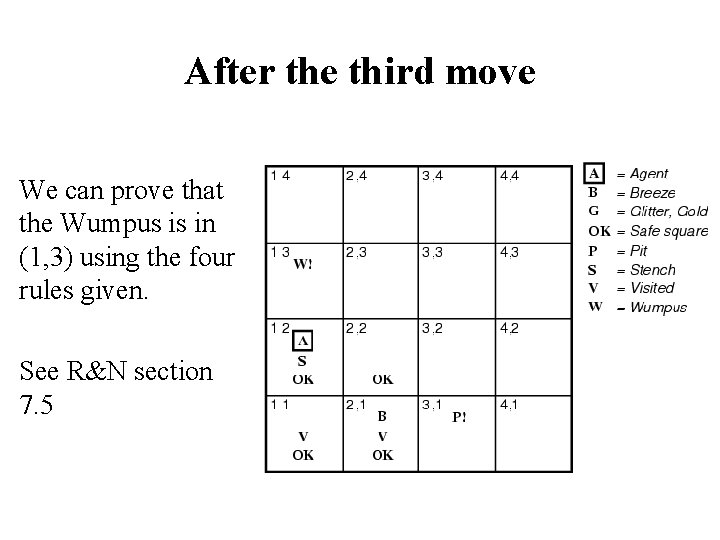After the third move We can prove that the Wumpus is in (1, 3) using the four rules given. See R&N section 7. 5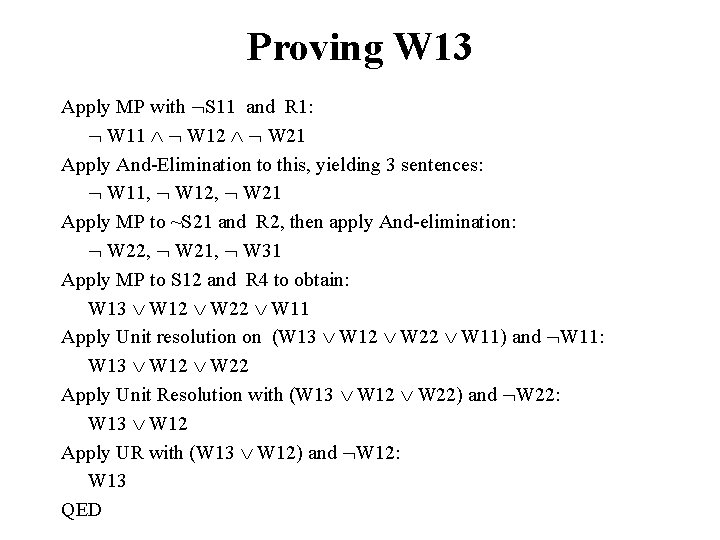Proving W 13 Apply MP with S 11 and R 1: W 11 W 12 W 21 Apply And-Elimination to this, yielding 3 sentences: W 11, W 12, W 21 Apply MP to ~S 21 and R 2, then apply And-elimination: W 22, W 21, W 31 Apply MP to S 12 and R 4 to obtain: W 13 W 12 W 22 W 11 Apply Unit resolution on (W 13 W 12 W 22 W 11) and W 11: W 13 W 12 W 22 Apply Unit Resolution with (W 13 W 12 W 22) and W 22: W 13 W 12 Apply UR with (W 13 W 12) and W 12: W 13 QED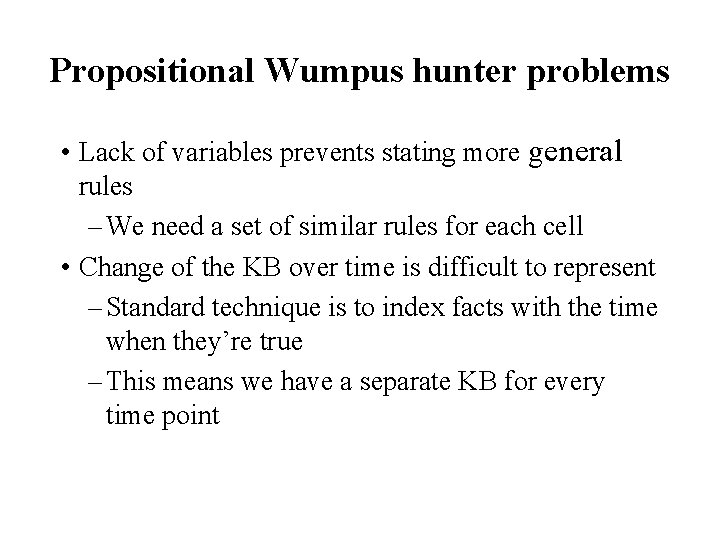Propositional Wumpus hunter problems • Lack of variables prevents stating more general rules – We need a set of similar rules for each cell • Change of the KB over time is difficult to represent – Standard technique is to index facts with the time when they’re true – This means we have a separate KB for every time pointPropositional logic summary • Inference is the process of deriving new sentences from old – Sound inference derives true conclusions given true premises – Complete inference derives all true conclusions from a set of premises • A valid sentence is true in all worlds under all interpretations • If an implication sentence can be shown to be valid, then— given its premise—its consequent can be derived • Different logics make different commitments about what the world is made of and what kind of beliefs we can have • Propositional logic commits only to the existence of facts that may or may not be the case in the world being represented – Simple syntax and semantics suffices to illustrate the process of inference – Propositional logic can become impractical, even for very small worlds